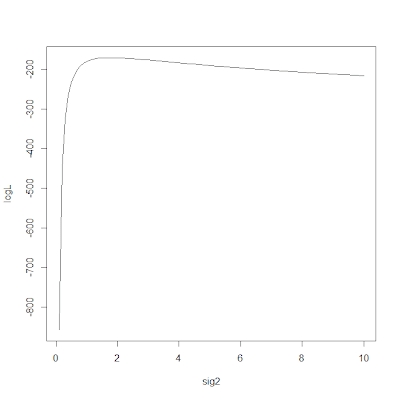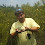## Wednesday, February 8, 2012

### New version of phylosig with control of multidimensional optimization migrated to the user

I just posted a new version of phylosig in which I have done two things to address the issue identified by Matt Johnson at Duke. He found that phylosig(...,method="lambda",se=se) failed to converge to the MLE of λ when the branches of the tree were rescaled to be much shorter in length (say to 1/1000 of their original length).

First, I changed the default starting values for ML optimization. In particular, for ML optimization of σ2 I had been using the REML estimate of σ2 conditioned on λ=1.0 and zero measurement error. (This is easy because this is just the mean square of the independent contrasts for the trait.) When we have lots of measurement error, this starting value puts us far past the MLE of σ2 for the full model. In addition, the log-likelihood surface is asymmetric around the MLE for σ2. This can be seen for the log-likelihood of the variance of a normal distribution via the following simple code:

> log.likelihood<-function(sig2,x,mu) sum(dnorm(x,mean=0,sd=sqrt(sig2),log=TRUE))
> x<-rnorm(n=100,sd=sqrt(2),mean=0)
> sig2<-1:100/10
> logL<-apply(as.matrix(sig2),1,log.likelihood,x=x,mu=0)
> plot(sig2,logL,"l")Second, I have also migrated several aspects of the control of the internally used R base package multidimensional optimizer, optim, to the phytools user. In particular, the user can now control the control parameters of optim as well as the starting values for optimization. Users may also notice that I now return the value for convergence (zero is good) & the message on convergence returned by optim.

Direct link to this updated code is here. Note that this only affects optimization of λ with within-species sampling error, because this is the only situation in which optim is used.

Let's see how these new updates affect our ability to solve our tricky tree & dataset from before.

> res1000<-phylosig(tree1000,xe,method="lambda",se=se)
> res1000
\$lambda
 0.7298903
\$sig2
 1037.687
\$logL
 -360.4223
\$convergence
 0
\$message
 "CONVERGENCE: REL_REDUCTION_OF_F <= FACTR*EPSMCH"

OK, well, that's pretty good. Let's try an even more dramatically rescaled tree:

> tree100000<-tree
> tree100000\$edge.length<-tree\$edge.length/100000
> res100000<-phylosig(tree100000,xe,method="lambda",se=se)
> res100000
\$lambda
 0.6357282
\$sig2
 51171.73
\$logL
 -364.529
\$convergence
 0
\$message
 "CONVERGENCE: REL_REDUCTION_OF_F <= FACTR*EPSMCH"

OK, that's not terribly far off the MLE of λ (which is better than before) but it's still not right. In addition, the function thinks that it has converged. Now, let's try and take advantage of the control parameters for optim.

> res100000<-phylosig(tree100000,xe,method="lambda",se=se, control=list(factr=1))
> res100000
\$lambda
 0.7298907
\$sig2
 103768.9
\$logL
 -360.4223
\$convergence
 0
\$message
 "CONVERGENCE: REL_REDUCTION_OF_F <= FACTR*EPSMCH"

This is cool. Here, I decreased the factor over machine precision that is used to control convergence. Setting it to 1.0 means that optim will only accept that it has converged when (to the maximum numerical precision of R) it can now longer improve the likelihood. Why, then, should we not just fix control=list(factr=1)? (The default is 107.) Well, for most datasets this would just add extra computation time (possibly a lot).

All right - try and break this version! (Just don't try too hard please.)

1.Thanks so much Liam! I have two comments/questions:

1) There appears to be a typo on line 126-- when test=T and me=T, convergence is spelled wrong so it always returns NULL when testing against lambda=0.

2) What are the kinds of values I should be using for "start"? I noticed that I needed to provide two numbers. The first number appears to be related to a random scaling of the squared mean of PIC for the trait on the tree, and the second is a random number between 0 and 1.

On my real data I would often get the "matrix is singular" error, but not every time I ran it! Certain combinations of the two values would work for some traits, while others would not.

Do you have any suggestions for improving these starting values so I can be assured that a trait does not run into this intermittent problem?

2.Hi Matt.

Issue #1 is indeed an error.

Issue #2 is that start should be a vector containing the starting values for ML optimization of sigma^2 and lambda. Thus, lambda should probably be on the interval of 0 to 1 (or the maximum value of lambda). And sigma^2 should be on the interval 0 to Inf, not including 0.

I have posted an updated version.

- Liam

3.Oh, and in addition the updates reject values of start that don't satisfy this criteria.

Note: due to the very large amount of spam, all comments are now automatically submitted for moderation.